This book is archived and will be removed July 6, 2022. Please use the updated version.

Electromagnetic Induction

# 88 Induced Electric Fields

### Learning Objectives

By the end of this section, you will be able to:

• Connect the relationship between an induced emf from Faraday’s law to an electric field, thereby showing that a changing magnetic flux creates an electric field
• Solve for the electric field based on a changing magnetic flux in time

The fact that emfs are induced in circuits implies that work is being done on the conduction electrons in the wires. What can possibly be the source of this work? We know that it’s neither a battery nor a magnetic field, for a battery does not have to be present in a circuit where current is induced, and magnetic fields never do work on moving charges. The answer is that the source of the work is an electric field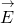that is induced in the wires. The work done byin moving a unit charge completely around a circuit is the induced emf ε; that is,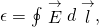where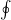represents the line integral around the circuit. Faraday’s law can be written in terms of the induced electric field as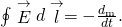There is an important distinction between the electric field induced by a changing magnetic field and the electrostatic field produced by a fixed charge distribution. Specifically, the induced electric field is nonconservative because it does net work in moving a charge over a closed path, whereas the electrostatic field is conservative and does no net work over a closed path. Hence, electric potential can be associated with the electrostatic field, but not with the induced field. The following equations represent the distinction between the two types of electric field: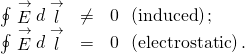Our results can be summarized by combining these equations: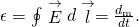Induced Electric Field in a Circular Coil What is the induced electric field in the circular coil of (Figure) (and (Figure)) at the three times indicated?

Strategy Using cylindrical symmetry, the electric field integral simplifies into the electric field times the circumference of a circle. Since we already know the induced emf, we can connect these two expressions by Faraday’s law to solve for the induced electric field.

Solution The induced electric field in the coil is constant in magnitude over the cylindrical surface, similar to how Ampere’s law problems with cylinders are solved. Sinceis tangent to the coil,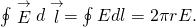When combined with (Figure), this gives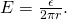The direction ofis counterclockwise, andcirculates in the same direction around the coil. The values of E are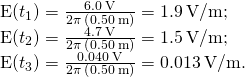Significance When the magnetic flux through a circuit changes, a nonconservative electric field is induced, which drives current through the circuit. But what happens if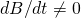in free space where there isn’t a conducting path? The answer is that this case can be treated as if a conducting path were present; that is, nonconservative electric fields are induced wherever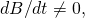whether or not there is a conducting path present.

These nonconservative electric fields always satisfy (Figure). For example, if the circular coil of (Figure) were removed, an electric field in free space at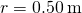would still be directed counterclockwise, and its magnitude would still be 1.9 V/m at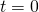, 1.5 V/m at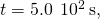etc. The existence of induced electric fields is certainly not restricted to wires in circuits.

Electric Field Induced by the Changing Magnetic Field of a Solenoid Part (a) of (Figure) shows a long solenoid with radius R and n turns per unit length; its current decreases with time according to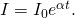What is the magnitude of the induced electric field at a point a distance r from the central axis of the solenoid (a) when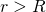and (b) when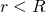[see part (b) of (Figure)]. (c) What is the direction of the induced field at both locations? Assume that the infinite-solenoid approximation is valid throughout the regions of interest.

(a) The current in a long solenoid is decreasing exponentially. (b) A cross-sectional view of the solenoid from its left end. The cross-section shown is near the middle of the solenoid. An electric field is induced both inside and outside the solenoid.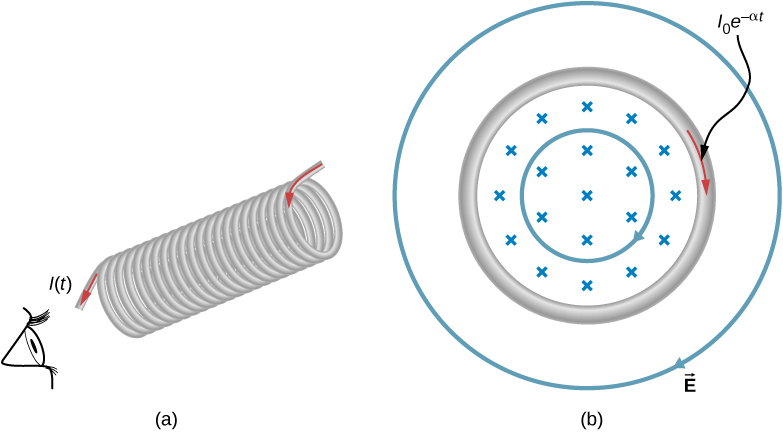Strategy Using the formula for the magnetic field inside an infinite solenoid and Faraday’s law, we calculate the induced emf. Since we have cylindrical symmetry, the electric field integral reduces to the electric field times the circumference of the integration path. Then we solve for the electric field.

Solution

1. The magnetic field is confined to the interior of the solenoid where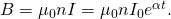Thus, the magnetic flux through a circular path whose radius r is greater than R, the solenoid radius, is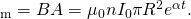The induced fieldis tangent to this path, and because of the cylindrical symmetry of the system, its magnitude is constant on the path. Hence, we have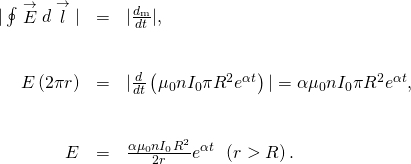2. For a path of radius r inside the solenoid,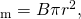so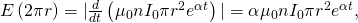and the induced field is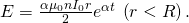3. The magnetic field points into the page as shown in part (b) and is decreasing. If either of the circular paths were occupied by conducting rings, the currents induced in them would circulate as shown, in conformity with Lenz’s law. The induced electric field must be so directed as well.

Significance In part (b), note that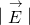increases with r inside and decreases as 1/r outside the solenoid, as shown in (Figure).

The electric field vs. distance r. When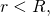the electric field rises linearly, whereas when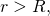the electric field falls of proportional to 1/r.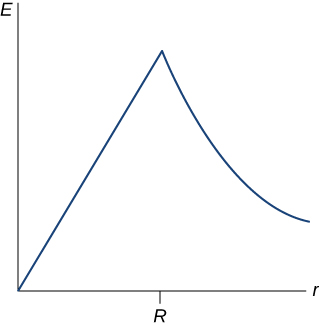Check Your Understanding Suppose that the coil of (Figure) is a square rather than circular. Can (Figure) be used to calculate (a) the induced emf and (b) the induced electric field?

a. yes; b. Yes; however there is a lack of symmetry between the electric field and coil, making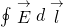a more complicated relationship that can’t be simplified as shown in the example.

Check Your Understanding What is the magnitude of the induced electric field in (Figure) atif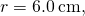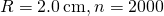turns per meter,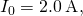and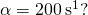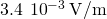Check Your Understanding The magnetic field shown below is confined to the cylindrical region shown and is changing with time. Identify those paths for which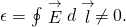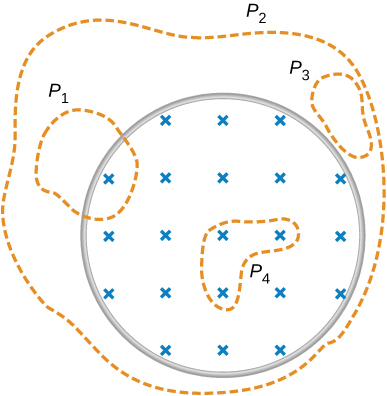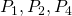Check Your Understanding A long solenoid of cross-sectional area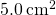is wound with 25 turns of wire per centimeter. It is placed in the middle of a closely wrapped coil of 10 turns and radius 25 cm, as shown below. (a) What is the emf induced in the coil when the current through the solenoid is decreasing at a rate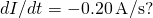(b) What is the electric field induced in the coil?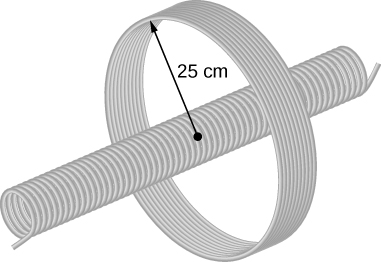a.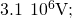b.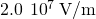### Summary

• A changing magnetic flux induces an electric field.
• Both the changing magnetic flux and the induced electric field are related to the induced emf from Faraday’s law.

### Conceptual Questions

Is the work required to accelerate a rod from rest to a speed v in a magnetic field greater than the final kinetic energy of the rod? Why?

The work is greater than the kinetic energy because it takes energy to counteract the induced emf.

The copper sheet shown below is partially in a magnetic field. When it is pulled to the right, a resisting force pulls it to the left. Explain. What happen if the sheet is pushed to the left?### Problems

Calculate the induced electric field in a 50-turn coil with a diameter of 15 cm that is placed in a spatially uniform magnetic field of magnitude 0.50 T so that the face of the coil and the magnetic field are perpendicular. This magnetic field is reduced to zero in 0.10 seconds. Assume that the magnetic field is cylindrically symmetric with respect to the central axis of the coil.

9.375 V/m

The magnetic field through a circular loop of radius 10.0 cm varies with time as shown in the accompanying figure. The field is perpendicular to the loop. Assuming cylindrical symmetry with respect to the central axis of the loop, plot the induced electric field in the loop as a function of time.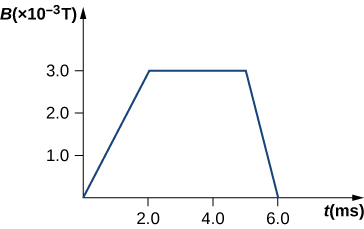The current I through a long solenoid with n turns per meter and radius R is changing with time as given by dI/dt. Calculate the induced electric field as a function of distance r from the central axis of the solenoid.

Inside,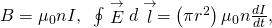so,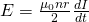(inside). Outside,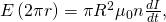so,(outside)

Calculate the electric field induced both inside and outside the solenoid of the preceding problem if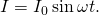Over a region of radius R, there is a spatially uniform magnetic field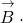(See below.) At,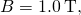after which it decreases at a constant rate to zero in 30 s. (a) What is the electric field in the regions where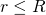and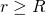during that 30-s interval? (b) Assume that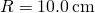. How much work is done by the electric field on a proton that is carried once clock wise around a circular path of radius 5.0 cm? (c) How much work is done by the electric field on a proton that is carried once counterclockwise around a circular path of any radius? (d) At the instant when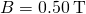, a proton enters the magnetic field at A, moving a velocity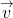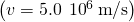as shown. What are the electric and magnetic forces on the proton at that instant?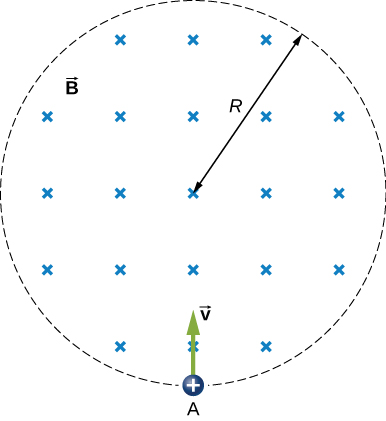a.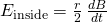,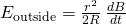; b.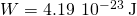; c. 0 J; d.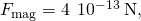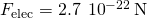The magnetic field at all points within the cylindrical region whose cross-section is indicated in the accompanying figure starts at 1.0 T and decreases uniformly to zero in 20 s. What is the electric field (both magnitude and direction) as a function of r, the distance from the geometric center of the region?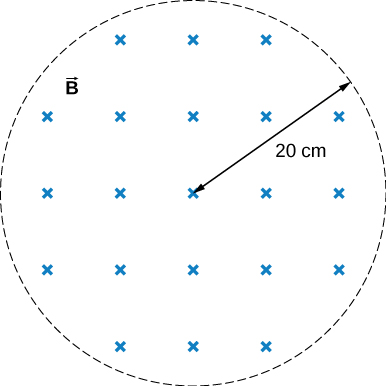The current in a long solenoid with 20 turns per centimeter of radius 3 cm is varied with time at a rate of 2 A/s. A circular loop of wire of radius 5 cm and resistance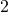surrounds the solenoid. Find the electrical current induced in the loop.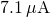The current in a long solenoid of radius 3 cm and 20 turns/cm is varied with time at a rate of 2 A/s. Find the electric field at a distance of 4 cm from the center of the solenoid.

### Glossary

induced electric field
created based on the changing magnetic flux with time